1.800.221.5175
Core Program
Full Access
Core Program
Full Access
High-Impact Tutoring
Core Program
Full Access
National Program
Full Access
New York
Critical Thinking for Active Math Minds
Preparing for Standards Based Assessments
Vocabulary Workshop, Tools for Comprehension Grades 1–5
Print Program
Interactive Edition
Print Program
Interactive Edition
Vocabulary Workshop, Tools for Excellence Grades 6–12+
Print Program
Interactive Edition
Vocabulary for Success
Progress English Language Arts Grades K–8
National Program
Full Access
High-Impact Tutoring
New York
Grammar Workshop, Tools for Writing
Grammar for Writing
Writing a Research Paper
Writing Workshop
Print Program
Interactive Practice Bundle
Fluency Booster Practice Book
Interactive Assessments
High-Impact Tutoring
Building Reading Success with Wiley Blevins
Print Program
Interactive Edition
Professional Learning

## How Math Games and Activities Increase Student Proficiency in Grade 4

Math games and activities can be an excellent tool to supplement and support math instruction. Using math games in your classroom allows students to practice mathematics in fun but also impactful ways. Students love games because they are engaging and exciting, and teachers love games because they help students practice what they've learned.

Grade 4 students extend their understanding of numbers up to 1,000 and gain additional knowledge of fractions, decimals, whole numbers, place value, and properties of operations. Through the use of number lines, fraction number lines, area models, counters, scales, dot paper, base-ten blocks, place-value charts, and place-value models, students will stay on target and have fun too.

## Divide with Number Squares

and Place Value

### Have students use number squares to divide.

To divide 73 by 2, students can model 73 in a place-value chart and then model sharing the squares equally between 2 sets, also shown in a place-value chart. As students develop understanding of the process, they can both explain their work and record it.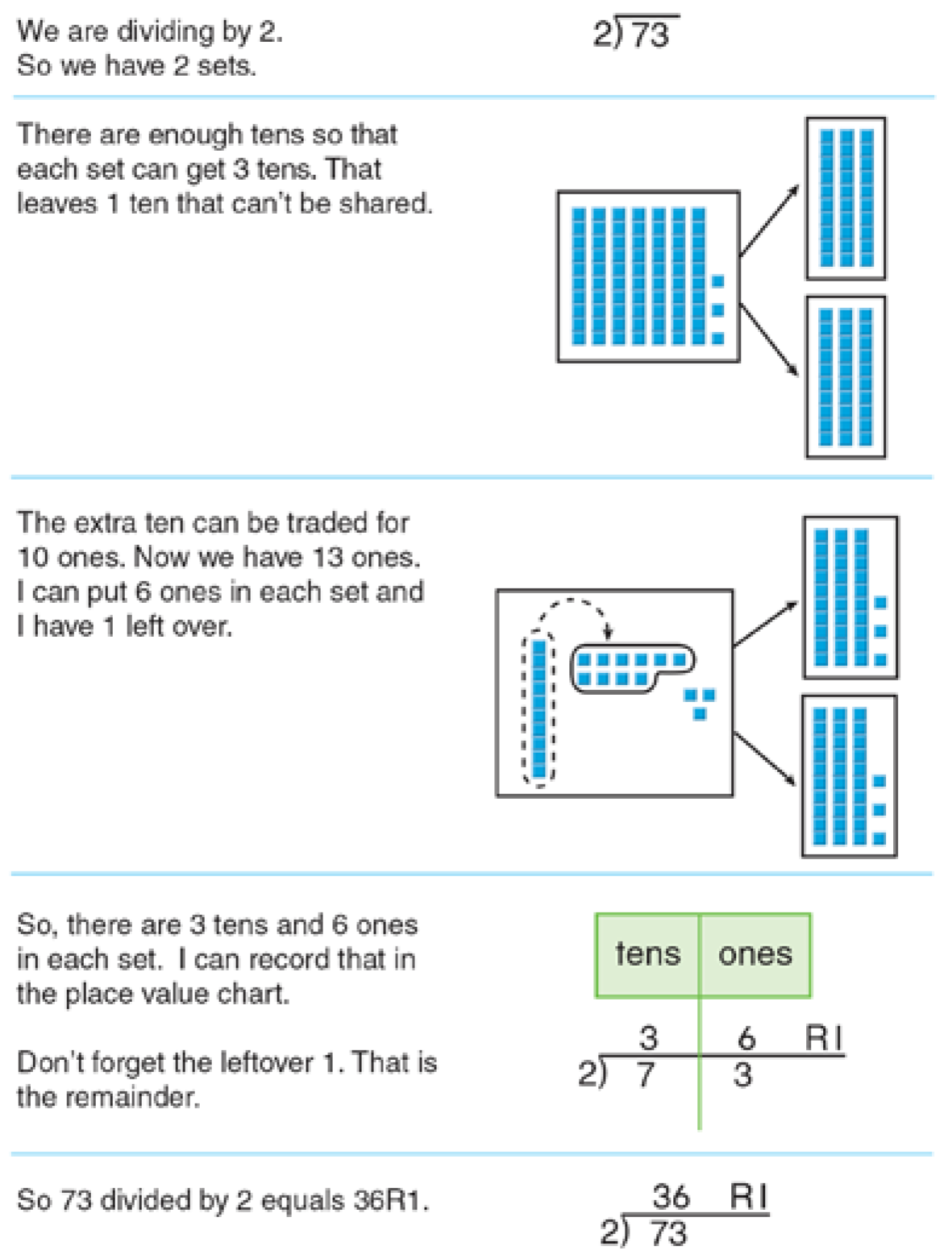Students who are able to model the division algorithm for a 2- or 3-digit number divided by a 1-digit number and translate their work into words and symbols will easily develop an understanding of the process and be able to apply it to any size whole-number dividends and divisors. They wil also have the necessry foundation for dividing decimals and integers.

References
Lampert, M. (1992) Teaching and learning long division for understanding in school. Analysis of arithmeic for mathematics teaching, 221-282, Hillsdale, NJ

## Fraction Number Line

### A fraction number line shows a unit fraction and its multiples. It helps students to see fractions as numbers and to understand their relationship to 1. Furthermore, a fraction number line helps students see relationships among fractions, including equivalency. The purpose of this instructional model is to facilitate students’ understanding of comparing fractions that have same or different denominators.

Students can first use a number line to compare fractions with the same denominators, just as they use a number line to compare whole numbers. On a number line, the number to the right is the greater number. Students can also see that, when the denominators of fractions are the same, they can simply compare the numerators.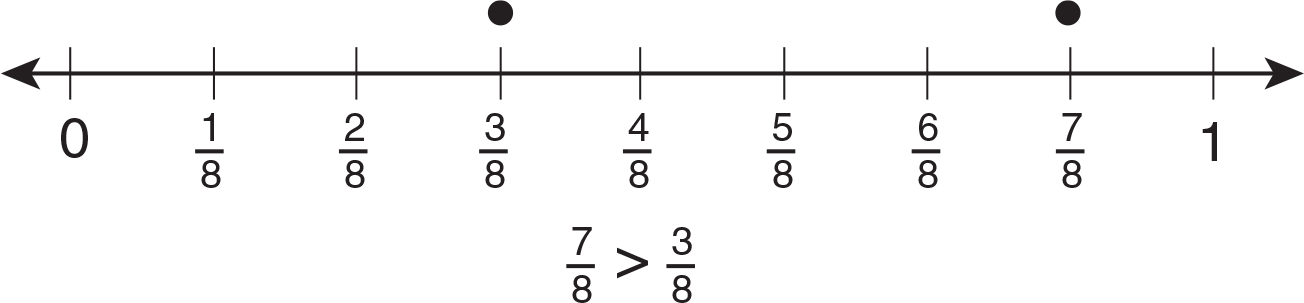Think: 78 is greater than 38 because 78 is to the right of 38 on the number line.
78 is also greater than 38 because 7 is greater than 3.

Students can next use a number line that shows equivalent fractions to find that, when fractions have different denominators, they cannot simply compare the numerators. They must first rename the fractions as equivalent fractions with the same denominators. Only then can they compare the numerators.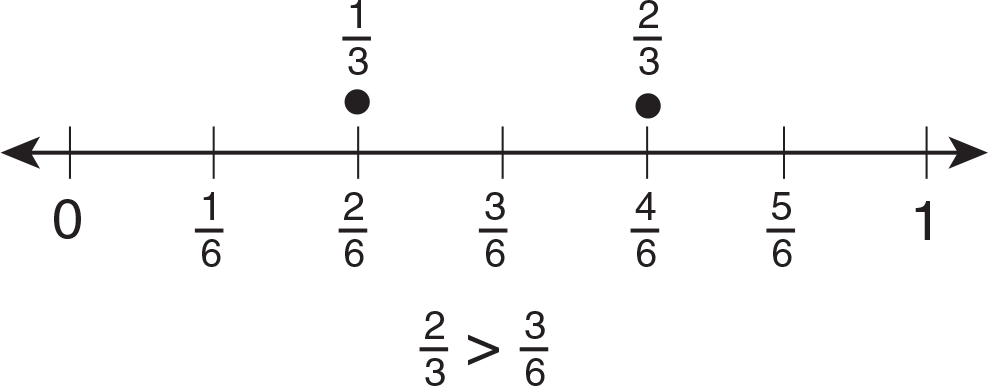Think: 23 is greater than 36 because 23 is to the right of 36 on the number line. The number line also shows that 23 is equivalent to 46 .  So, to compare 23 and 36 , you can compare 46 and 36 .  46 is greater than 36 because 4 is greater than 3.  So, 23 is greater than 36 .

Students who understand how to use a number line to compare fractions with different denominators will understand the steps needed to compare and order fractions without the use of concrete materials or pictures, as well as the steps needed to add and subtract fractions with different denominators.

References
Burns, M. (2000), About Teaching Mathematics: A K-8 Resource, 223–237, Math Solutions Publications, Sausalito, CA.

## Hands-On Approach

### Many recent studies suggest that hands-on experiences which allow for the use of technology may facilitate students’ data organization and graphing skills. The instruction model presented here is an example of using a hands-on approach, which may help students to choose an appropriate graph.

In the following exercise, students are to determine whether creating a bar graph or a line graph will better show the given data.

Step 1: Assign students to groups of 3 or 4. Have each group choose a topic to survey (for example: favorite food or sports team), and ask them to collect their data over the course of one school day.

Step 2: Next, ask groups to organize their survey data, and create both a bar graph and a line graph. Have each group share with the class their survey results along with the graphs they created. Ask: “Which graph better displays the information gathered?”

Step 3: Using technology, have each group create a bar graph and a line graph that shows this specific example: “A school’s student population changes over 50 years. In 1957, 110 pupils were enrolled. In 2007, 820 pupils were enrolled.”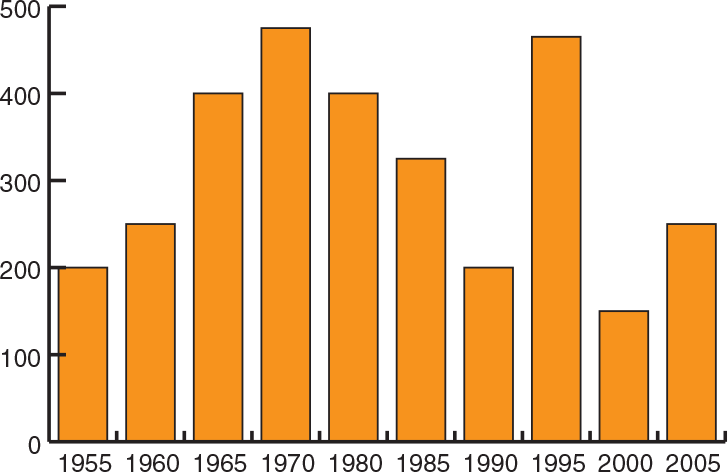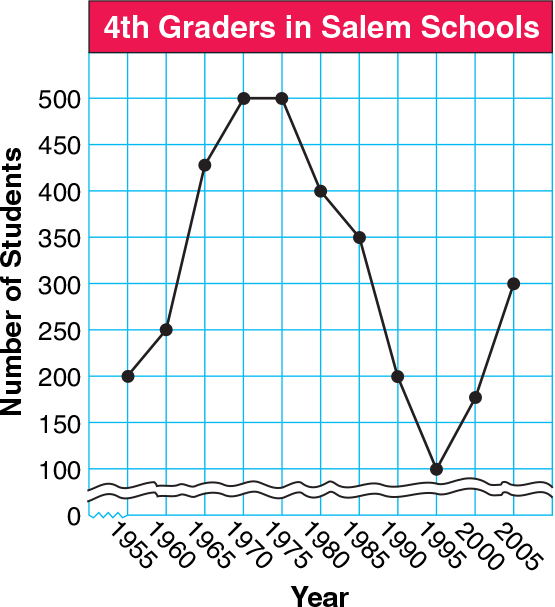Allow independent time for each group to create these graphs, but actively discuss with them what the horizontal and vertical axes will look like. For example, ask students to consider whether a scale in hundreds would be helpful here.

After the graphs are finshed ask, “Which graph better shows the change in student population over time, and why?” Utilization of both hands-on and technology experiences should allow students to see and understand that the line graph more effectively represents the change over time, while the bar graph better shows the data being compared. Encourage groups to discuss and write down all the differences they observe in the two types of graphs.

The use of technology as a learning tool can provide students with multiple options for interactive production and manipulation of graphic displays. For example, they may change the scale of the axes quicker than if created by hand. However, it is best to keep the focus on activities that actively engage students in the collecting of information, brainstorming the best way to display those findings, and then actively creating a graph. This will better reinforce the conceptual understanding of statistics and probability topics.

References
J.A. Van De Walle Elementary and Middle School Mathematics: Teaching Developmentally, Addison-Wesley Longman, Boston, MA (2001).

## Number Line

### In Lindquist’s study, he suggested that students need to understand that each measurement unit can be partitioned into smaller parts. Lehrer’s research suggested that many students respond to linear measurement with a non-zero origin by simply reading off whichever number on a ruler aligns with the object. Working with a number line can help students acquire the skills they need to be able to read the variety of scales found on real-world measuring tools.

Students are often asked to measure to the nearest 12 inch or 14 inch. Students can work with a fraction number line, rounding the fractions and mixed numbers marked with points to the nearest 12 and 14 .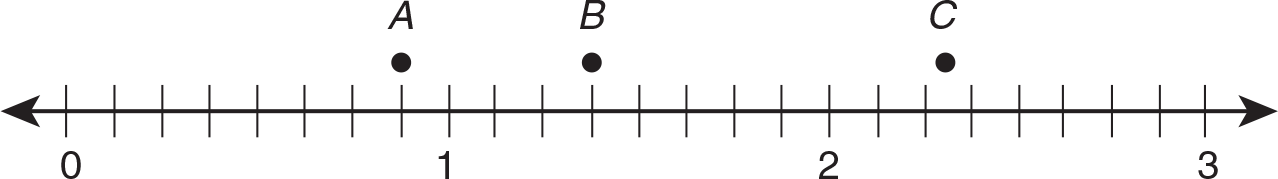Students can work with a decimal number line in preparation for rounding to the nearest centimeter.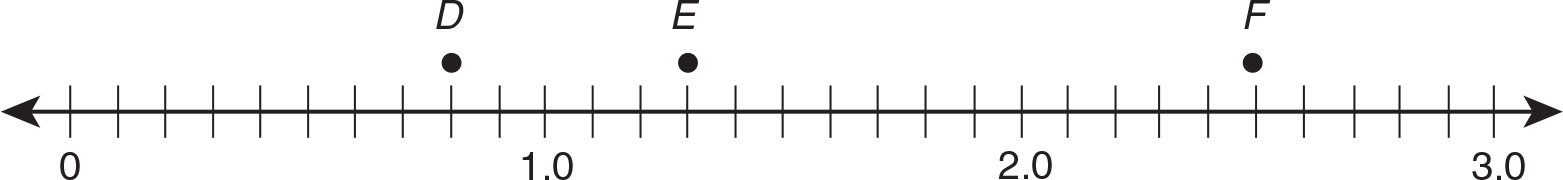D is 0.8 and rounds to 1.
E is 1.3 and rounds to 1.
F is 2.5 and rounds to 3.

A number line with marks for even numbers and labels for tens can prepare students for reading scales on thermometers.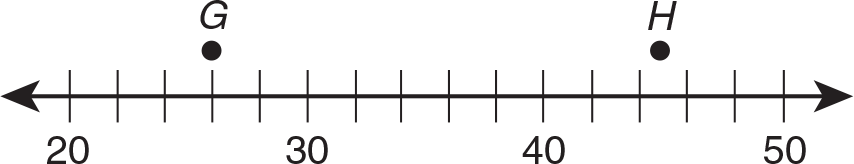G is 26, and H is 45.

Students who can easily name points and round numbers on a number line will be able to apply these skills to using measurement tools.

References
Lindquist, M. (1998) The measurement standards. The Arithmetic Teacher, 37, 22–26
Lehrer, R., Jenkins, M., & Osana, H. (1998) Longitudinal study of children’s reasoning about space and geometry. Designing learning environments for developing understanding of geometry and space, 137–167, Mahwah, NJ: Erlbaum

## Part-Part-Whole Mat

### Many research studies suggest that students best develop their early algebraic thinking through concrete activities and real-world problem situations. The following instructional model demonstrates a hands-on activity to help students conceptualize the inverse relationship between addition and subtraction and further develop ideas of variables and expressions.

Read or display this problem: Matt has 5 tennis balls in his backpack. He left the rest of his tennis balls at home. He has 12 tennis balls in all. How many tennis balls did Matt leave at home?

Students can model this problem using counters on a part-part-whole mat or can repre- sent their work symbolically on a part-part-whole mat.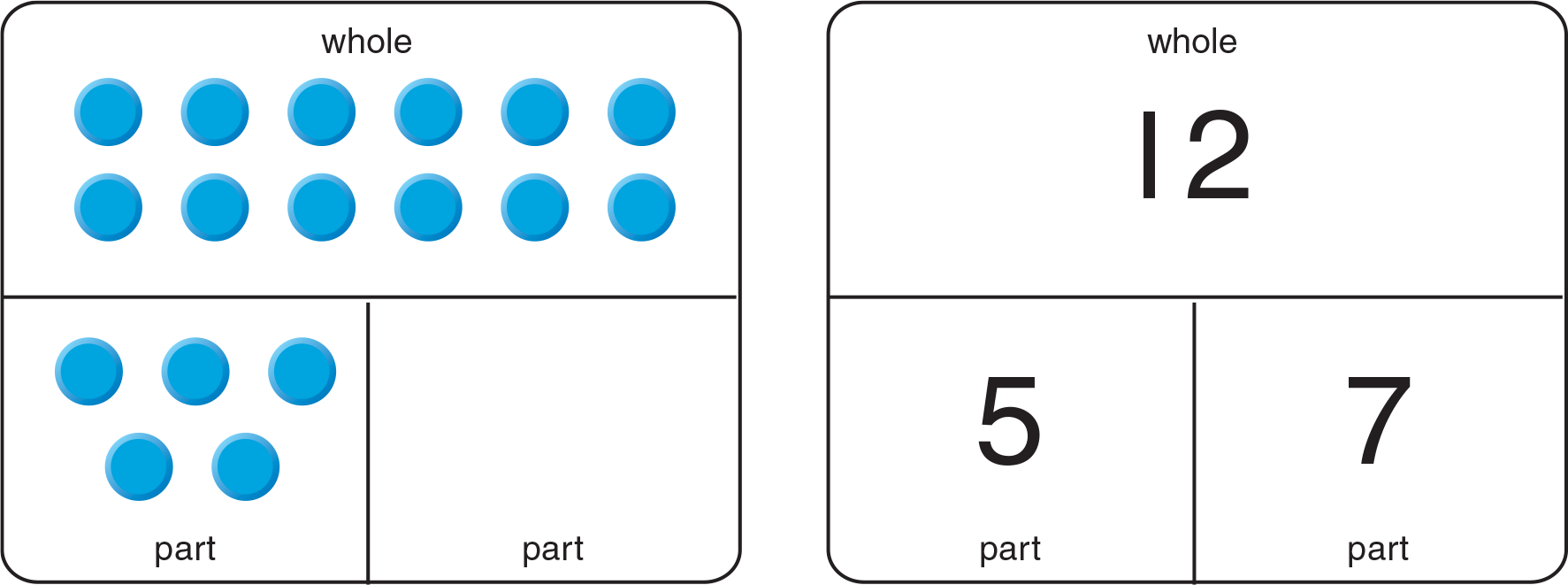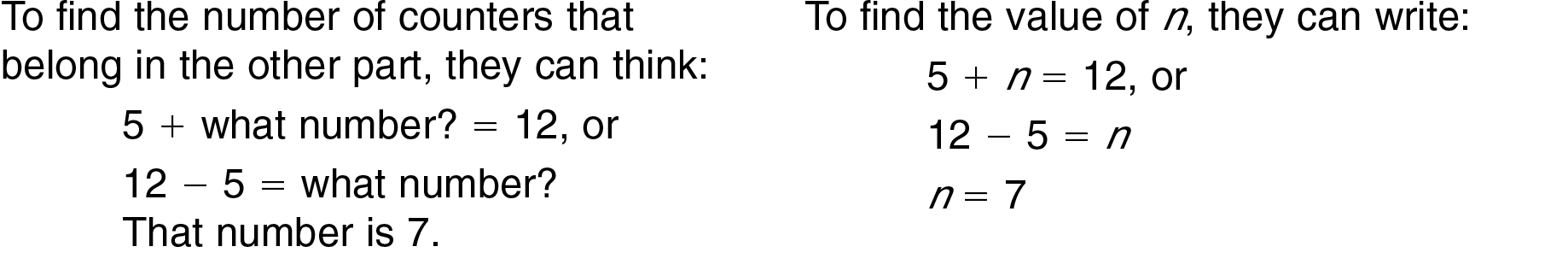To check this problem, students can put 7 counters in the empty part and count to verify that the number of counters in both parts equal the number of counters in the whole. Or, students can replace n in the mat with 7 and add to verify that the sum of the numbers in the parts equals the number in the whole.

References
Van de Walle, J. A. (1998). Elementary and Middle School Mathematics: Teaching Developmentally, 55, 401 New York, NY

## Area Model

Multiplication

### An area model drawn on grid paper is closely related to an array and is also a powerful representation of the muliplication algorithm.1

The grid below shows the area of a 12 by 13 rectangle. It also models how the product of 12 × 13 is the sum of four partial products by demonstrating the application of place value concepts and basic multiplication facts leading to a deeper understanding of the Distributive Property of Multiplication over Addition.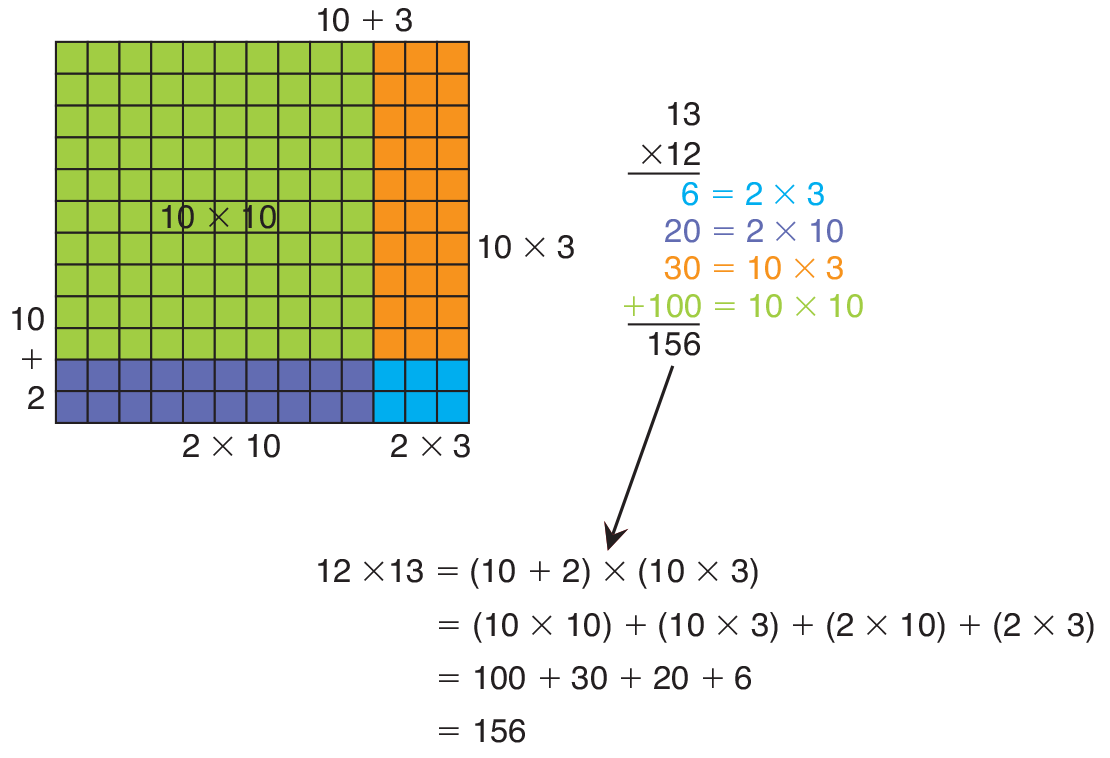Understanding of this model and its relationship to the Distributive Property of Multiplication over Addition leads to the use of the expanded multiplication algorithm as shown above.

Students who understand what each partial product represents in this expanded algorithm will be able to easily understand that the traditional multiplication algorithm is simply a shortcut.1 National Research Council. (2001). Adding It Up: Helping Children Learn Mathematics, 181–229.

## Area Model

Division

### The following instructional model is adapted from an example presented in Adding It Up. Because of the size of the numbers, it is difficult to use concrete materials to model multidigit division. However, students can build this area model "from the top down" as they subtract equal to the divisor from the dividend.

To decide how many groups to subtract each time, students should estimate the number of groups that they should subtract in a variation of the Guess and Test strategy. They test  this by multiplying and subtracting. If more groups can be subtracted, they estimate how many more, and continue in this manner until the number of groups that remains is less than the divisor.

By breaking the dividend and divisor into multiples of 10 with 5 left over, students can use the following area model to continue subtracting multiples of 45 (40 and 5) until the remainder is less than 45. This method also builds experience with estimating because students multiply by the correct number (50, then 10, then 2, then 1) as they write each partial quotient.

Find 2,846 ÷ 45.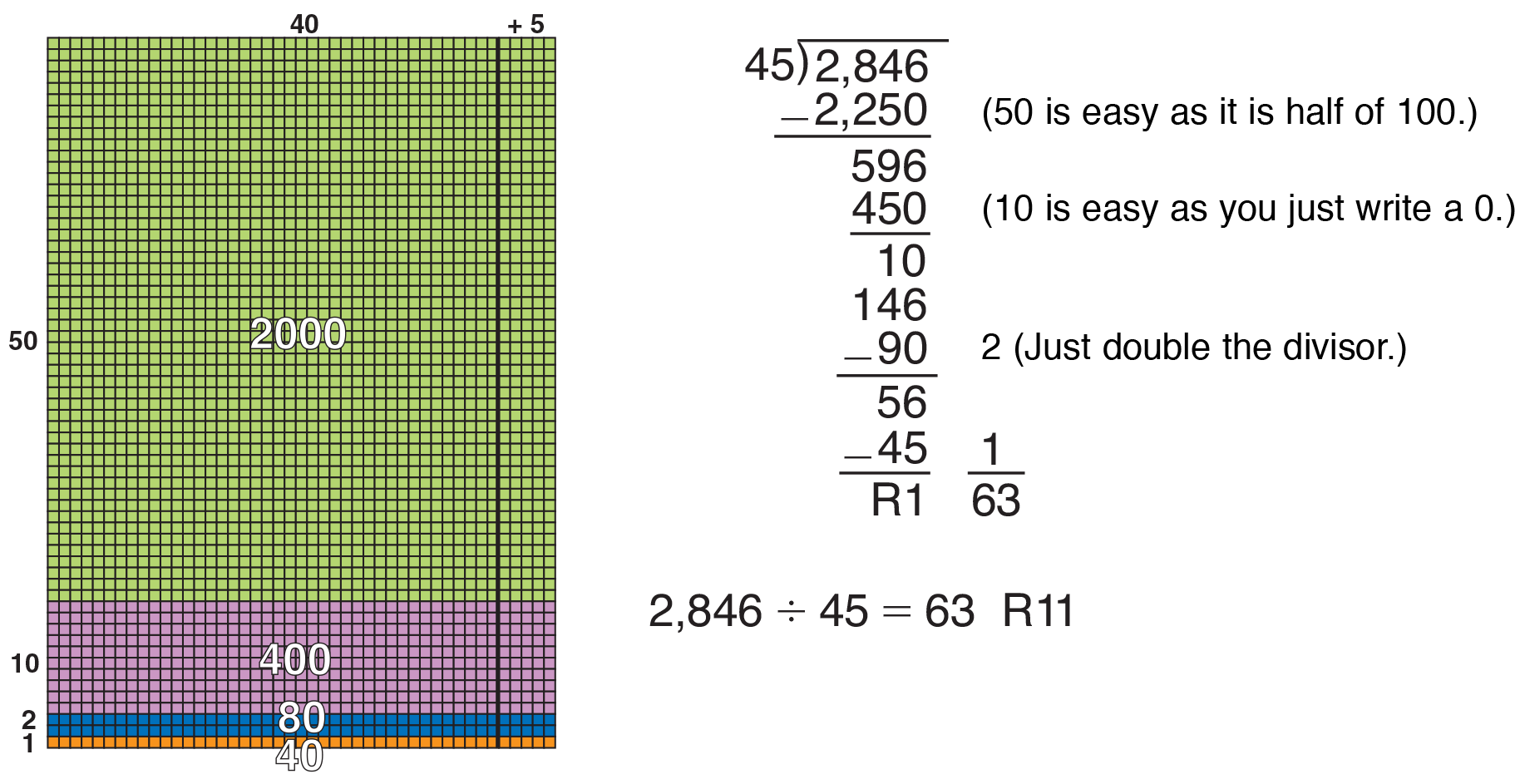Students who understand this work can record it in the standard division algorithm. Because they were able to subtract 50 + 10 (60), groups of 45 from 2,846, they can begin writing the quotient above the division house. That is, they can write 6 in the tens place and continue from there.

References
Van de Walle, J. A. (1998). Elementary and Middle School Mathematics: Teaching Developmentally, 55, 401 New York, NY.
National Research Council, (2001). Adding It Up: Helping Children Learn Mathematics, 181–229.
Lampert, M. (1992) Teaching and learning long division for understanding in school. The Analysis of Arithmetic for Mathematics Teaching, 221–282, Hillsdale, NJ.

## Base-Ten Blocks

### Base-ten blocks can be useful in helping students make the link between whole-number and decimal numeration. Begin by reviewing the basis of whole-number place value— that there is a 10-to-1 relationship between any two adjacent places, and that, moving from right to left, each place is 10 times the previous place. In contrast, moving from left to right, each place is one tenth smaller than the previous place. (When using whole numbers, the unit cube stands for a one.)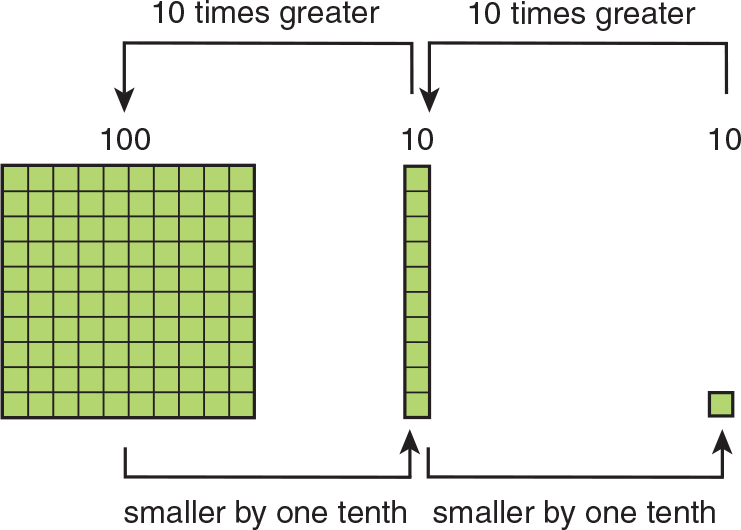Demonstrate that the same relationship exists between any two adjacent places in a decimal. This time, the flat stands for a one.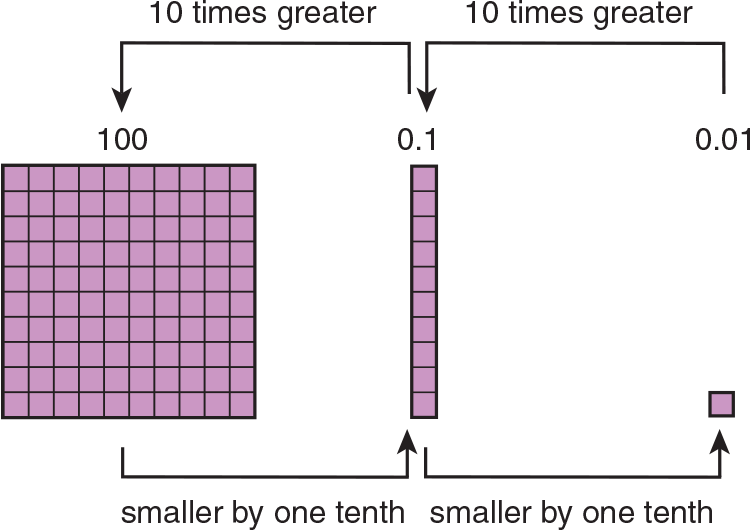Whether modeling whole-number place value or decimal place value, guide students to see that the 10-to-1 relationship continues indefinitely to the left or to the right. Students are only limited—for the purposes of modeling—by the base-ten blocks available.

Students who understand the basis of decimal place value will more easily be able to apply what they know about ordering and computing with whole numbers to their work with decimals.

Students can also use base-ten blocks along with decimal squares to make the work of representing decimals less tedious. Instead of coloring the decimal squares, students may place flats, rods, and units on the squares.

References
Stix, A. (1997) Teaching Fractions and Decimals: Fun with Picture Grids. ERIC ED408158

## Cups, Counters, and Balance Scale

### Much research suggests that students best develop their early algebraic thinking through concrete activities. Cups and counters on a balance scale are useful tools to help students solve equations and understand and make function tables.

To find the value of a variable in an equation such as 3a = 6 + 6, have students place three small plastic cups, each labeled a, on the left side of a balance scale, and 2 stacks of 6 counters each on the right side.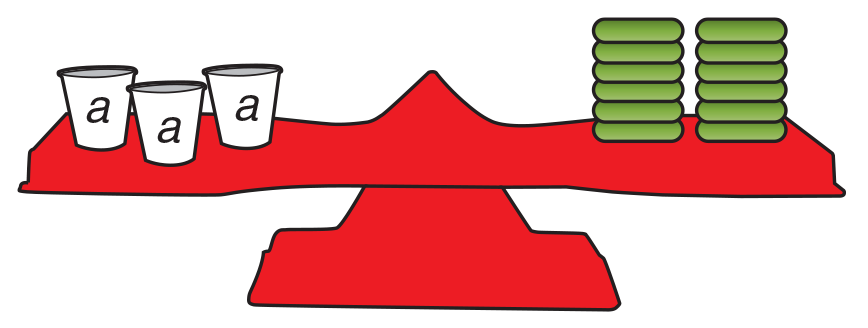To find the value of a, students need to determine how many counters to put in each cup so that both sides balance. Students can use the Guess and Test strategy or they can divide. There are 12 counters on the right side (6 + 6), so there must be 12 counters on the left side, which must be evenly distributed among the 3 cups. Students divide 12 by 3, and conclude that they should put 4 counters in each cup. Students place the counters in the cups to check this answer. Finally, they can show their findings symbolically.To model a function such as y = x + 3, students can place a cup labeled y on the left side of the scale and a cup labeled x and 3 counters on the right side of the scale.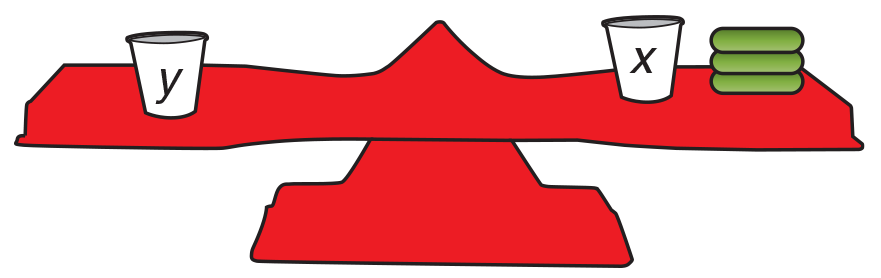To represent different values of x, students can put different numbers of counters in the cup labeled x. Then, they can find how many they must put in the cup labeled y for the scale to balance. Finally, they can represent their work in a function table.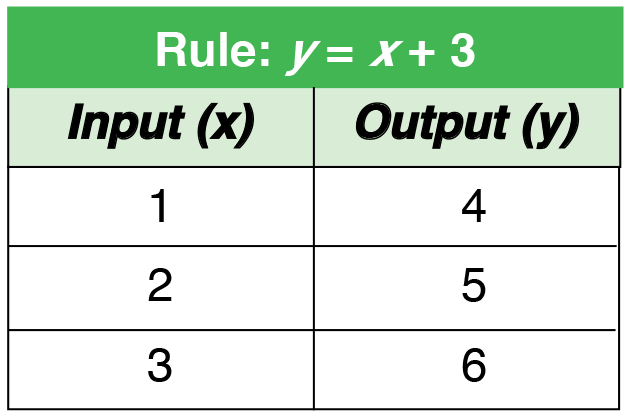Students who can use cups, counters, and a balance scale should develop a good understanding that a variable may represent one or more values, that both sides of an equation must be equal, and that y-values in a function depend on the corresponding x-values.

References
Van de Walle, J. A. (1998). Elementary and Middle School Mathematics: Teaching Developmentally, 55, 401 New York, NY
Carpenter, T. P.; Franke, M. L.; Levi, L. (2003) Thinking Mathematically: Integrating Algebra and Arithmetic Elementary School. Portsmouth, NH. Heinemann.

## Dot Paper

### Understanding solid figures requires an even greater ability to visualize spatial relationships than does the understanding of plane figures. Dot paper is especially useful for exploring these spatial relationships, as parallel lines and different angles can be easily drawn.

Square dot paper can be used to design nets; flat patterns that fold into solid figures. You can provide students with the net for a certain figure, such as Net A below, which forms a rectangular prism. After students cut out the nets, demonstrate how to fold and tape them together.

Have students name the plane figures that are the faces of the solid figure. Then have students design other nets to make the same plane figure and test them. For example, Net B can also be folded to make a rectangular prism, but Net C cannot. This work will help students understand which shapes the faces must be and where they connect.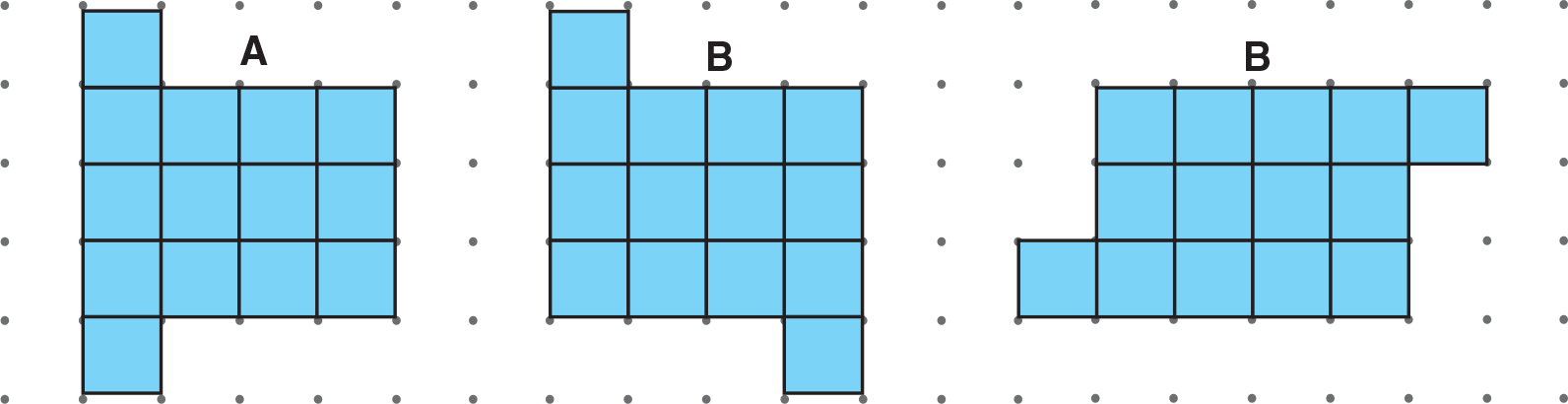Triangular dot paper can be used to draw solid figures, which will help students understand how 3-dimensional figures can be represented in two dimensions, as they appear in their textbooks. First, have students build solid figures out of connecting cubes. Then, have them copy these figures onto triangular dot paper. Before students begin, demonstrate how to draw a single cube and then a very simple rectangular prism. They can then move on to more complicated figures.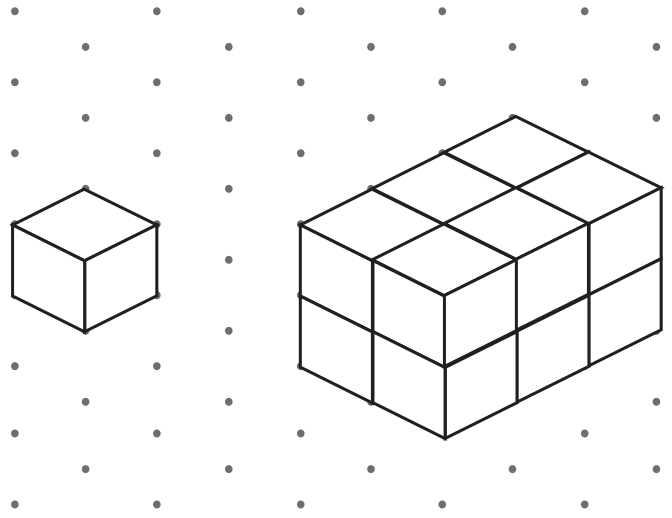This skill will also help students to apply the problem-solving strategy Draw a Picture, featured in this chapter.

References
Rahn, James (2005) Geometry Problems? Help Students Prepare for the Geometry and Measurement Standard on the HSPSA. Rutgers University, Piscataway, NJ.

## Fraction Strips

### The purpose of this instructional model is to help students develop conceptual understanding of adding and subtracting fractions. Students who can visualize what the numerator and denominator of a fraction represent and can write fractions for models are able to more readily understand why the numerators are added and subtracted and why the denominator remains the same. Such students can also understand why fractions with unlike denominators can and must be renamed in order to be added or subtracted.

Begin by distributing fraction strips. Have students paste two fraction strips, like the ones below, onto a sheet of paper to model 38 + 18 .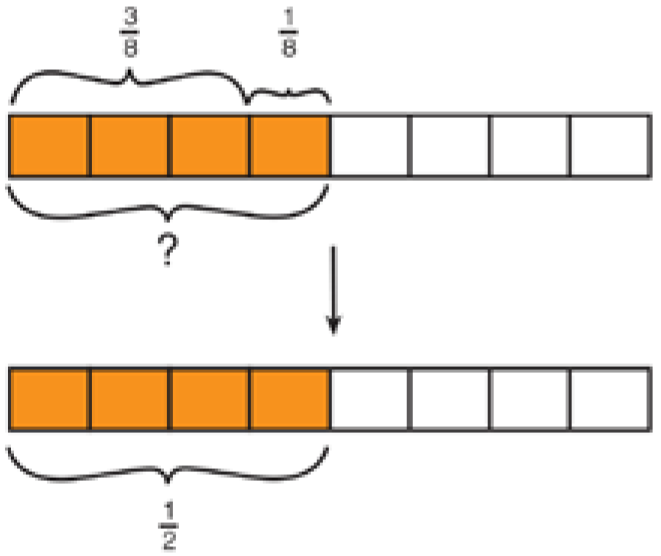Point out the relationship between 38 + 18 , shown by the upper fraction strip, and "?", shown by the lower fraction strip. (The two strips show the same size.) Have students record the sum, "?", below their models. Students may question why 38 + 18 does not equal 48 . Point out that 48 and "?" are equivalent, and remind students that "?" is 48 in its simplest form.

Continue in this manner with the following models to demonstrate subtraction with like denominators.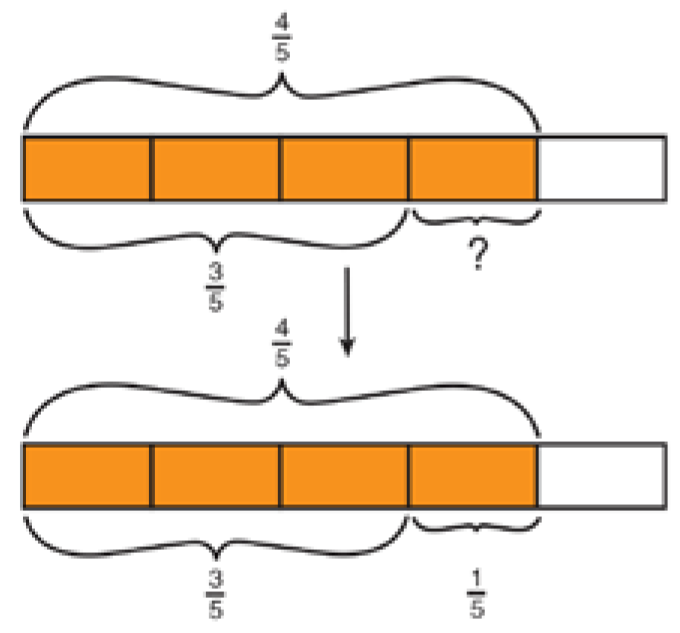References
Van de Walle, J.A. (1999). Elementary and Middle School Mathematics: Teaching Developmentally, 55, 401 New Yok, NY

## Models and Place-Value Charts

### Various research findings suggest that sustained experiences with concrete models are needed for students to connect discrete quantities with symbolic representations. Used along with place-value charts, they help students develop analogical reasoning of place value of greater numbers. Before beginning this activity, work with students to cut several small squares out of tagboard. Label the squares: 100,000,000;  10,000,000;  1,000,000;  100,000;  10,000;  1,000;  100;  10;  and 1. Then, use the labeled squares and a place-value chart to model a number such as 210,234,630. (In this chart, a key was used for space considerations.)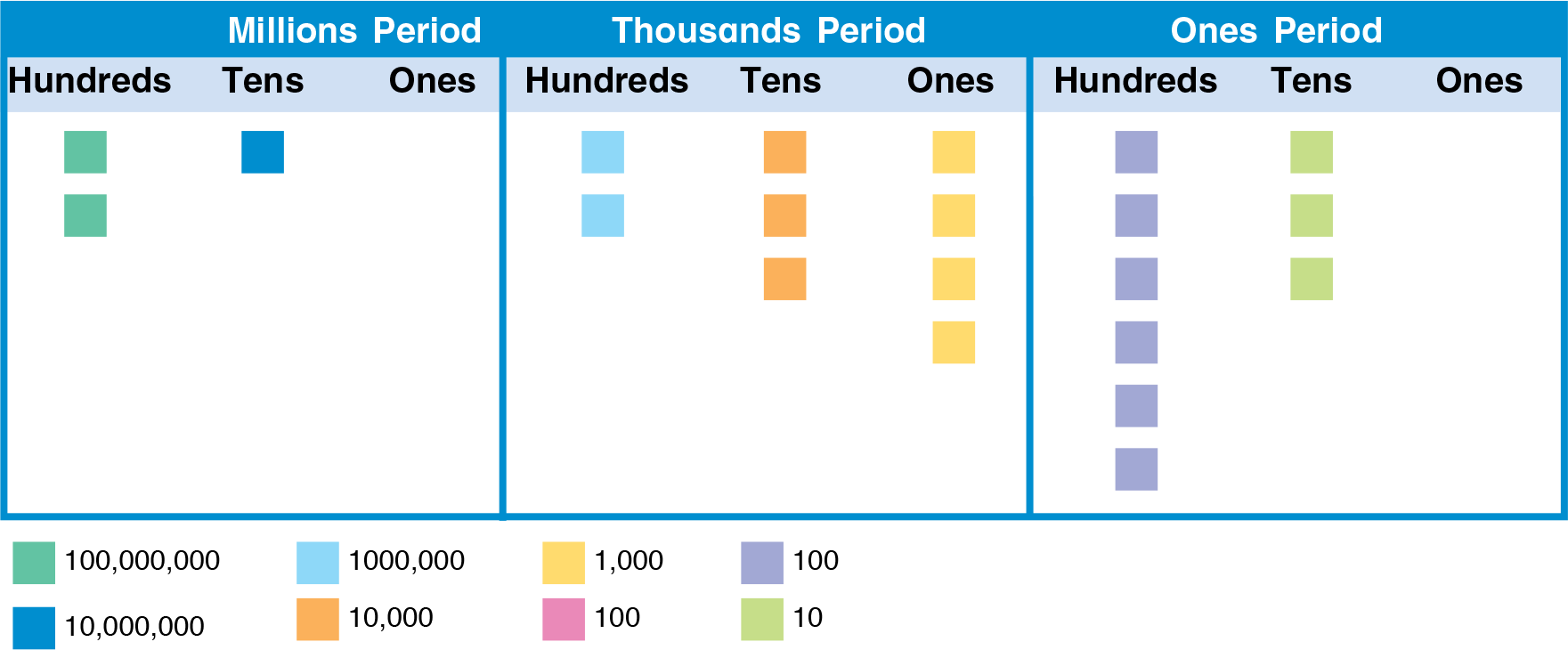By working from left to right and counting the squares in each column of the chart, students can also write the standard form of the number modeled. There are 2 squares in the hundred millions column, 1 square in the ten millions column, 0 squares in the one millions column, and so on. So, the standard form of the number is 210,234,630. By working from left to right and writing the value of the squares in each column, students can write the expanded form of the number modeled: 200,000,000 + 10,000,000 + 200,000 + 30,000 + 4,000 + 600 + 30.

Students can also use their number squares in a place-value chart to model counting on and counting back by 10s, 100s, and 1000s. Have them model a number such as 45,832 in the chart. To count on by 10s, they can repeatedly add one 10 square in the tens column and say the new numbers shown: 45,842;  45,852;  45,862. To count back by thousands, they can repeatedly remove one 1,000 square from the thousands column and say the new numbers shown: 44,832;  43,832;  42,832.

At later levels, as place-value concepts are extended to very great and very small numbers, including the use of scientific notation, the abstraction of the numbers and their symbolic representations increase. Modeling of place-value concepts at this level and earlier will strengthen students’ grasp of basic place-value concepts and prepare them for more advanced work.

References
Varelas, M. & Becker, J. Children's Developing Understanding of Place Value: Semiotic Aspects. Cognition and Instruction, v15 n2, 265–286, 1997 Bove, S. P., Place Value: A Vertical Perspective. Teaching Children Mathematics, v1 n9, 542–46, May 1995

## Number Squares and Place-Value Charts

### The following instructional model utilizes the number squares that children created for the activity developed for Chapter 1 and place-value charts to depict the process of regrouping in addition and subtraction algorithms. Before attempting to model addition or subtraction with their squares, students must understand that only squares with like numbers on them can be added or subtracted. Therefore, they may need to trade ten ones squares for one 10 square or vice versa.

Example A: Model 4,526 + 2,135.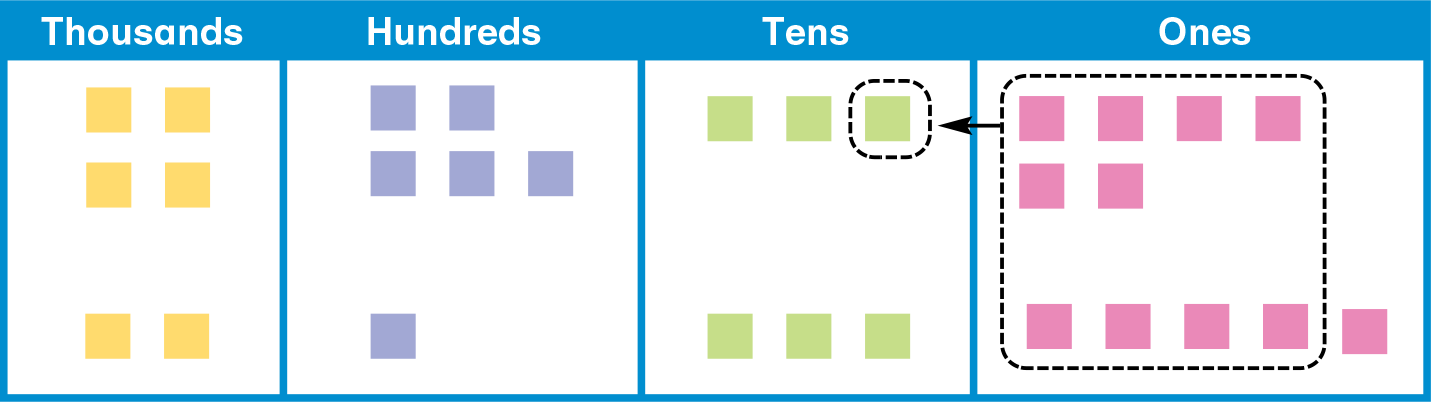As students model adding from right to left, regrouping as needed, have them explain their work and record it. The recording is shown below by steps, but students can show all the steps, one at a time, with one set of addends.Example B: Model 4,178 − 2,543.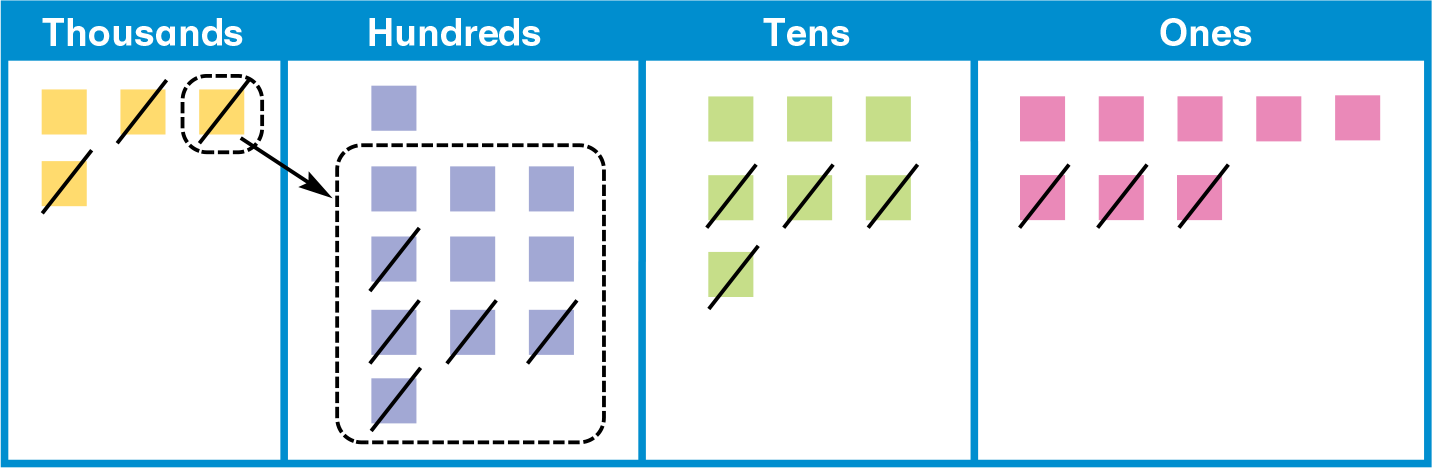Students who are able to model addition and subtraction with regrouping and to translate their work into words and symbols will easily develop the understanding of place-value concepts that underlie addition and subtraction with any size whole numbers and any numbers of regroupings.

References
National Research Council, (2001). Adding It Up: Helping Children Learn Mathematics, 181–229
Fiori, C. & Zuccheri, L. (2005) An Experimental Research on Error Patterns in Written Subtraction. Educational Studies in Mathematics, v60 n3 p323-331 Nov 2005

## Geoboard Manipulative

### Many research studies suggest that the use of a geoboard can facilitate students’ spatial sense and geometry skills. The instruction model presented here is an example of how to use a geoboard manipulative to work towards this goal.

To investigate the different types of triangles, students can use the geoboard’s rubber bands to construct a variety of three-sided possibilities. Students can estimate the measures of the sides and angles when designing the figures, but final verification must be measured with a ruler or a protractor, and finally recorded in a table for further investigation.

• Have students design the three different triangles below.
(A) A triangle with no equal sides.
(B) A triangle with a 90-degree angle.
(C) A triangle with opposite equal angles.
• Once constructed, students must use a ruler and a protractor to verify that the triangle they made matches the descriptions above.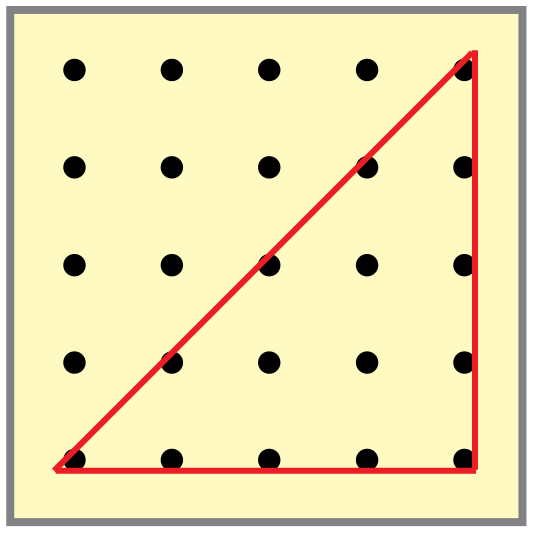• Students should then record all their measurements and observations in the given table. For those who finish early, allow them to recreate one of their triangles with the same angle measures, but different side lengths.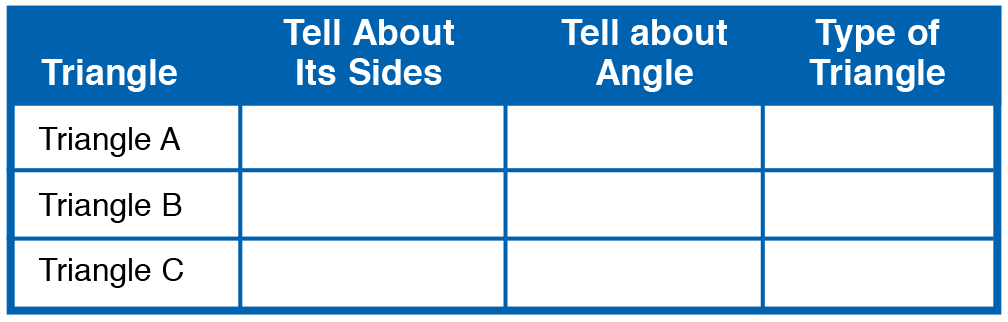As students share their findings, you can introduce the names of the different types of triangles.

Encourage students to use the geoboard to further explore the construction of a variety of triangle types not created in the activity. You can also challenge students to find the sum of the measures of the angles of any triangle.

Repeat these activities with quadrilaterals. Besides finding the measures of the angles and sides of the figures, students should also note whether any sides are parallel or perpendicular.

References
Burns, M. (2000), About Teaching Mathematics: A K-8 Approach, Math Solutions Publications, Sausalito, CA.